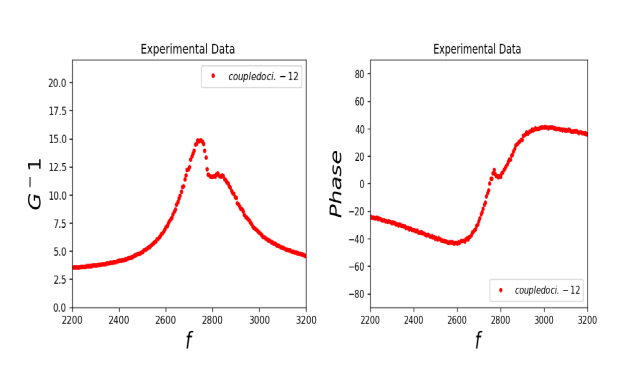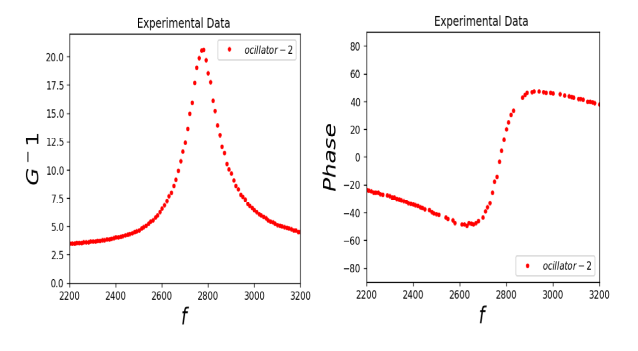# Frequency and Phase Response of Oscillators

## Study the frequency and phase response of coupled and uncoupled oscillators via Python Code and ExpEYESBrief Summary
A student submitted Project that uses python code to apply various input frequencies to oscillators, and measure the output waveform and extract parameters such as frequency, amplitude and phase. Coupled and uncoupled oscillators have been studied

## Introduction

### Bode plots

Procedure to characterise oscillators

• Connect WG to the input of the circuit, and also to A1
• Connect the output of the circuit to A2
• Start logging data via the Python code below.

## Results from coupled and uncoupled oscillators## Write Python Code

Data collection for this experiment is via this Python Code.

``````from pylab import *
from scipy import optimize
import csv
import time
def sineFunc(x, a1, a2, a3, a4):
return a4 + a1*sin(abs(a2*(2*pi))*x + a3)

with open('mycsv.csv', 'r') as csv_file:
with open('set1.1.csv','w') as new_file:
fieldnames = ['freq','gain','phase']
csv_writer =csv.DictWriter(new_file,fieldnames =fieldnames ,delimiter=',')
f = 1500
while f < 5000:
if f == 4000:
break
f += 10
p.set_sine(f)
time.sleep(0.1)
t,v, tt,vv = p.capture2(1000, 20)   # captures A1 and A2
f1 = [1, f, 0.0, 0.0]   # Amp, freq, phase , offset
f1, pcov = optimize.curve_fit(sineFunc, t*0.001, v, f1)
f2 = [1, f, 0.0, 0.0]   # Amp, freq, phase , offset
f2, pcov = optimize.curve_fit(sineFunc, tt*0.001, vv, f2)
gain = abs(f1/f2)
dphi = (f2 - f1) * 180/ pi
csv_writer.writerow({'freq':f, 'gain':gain, 'phase':dphi})

``````